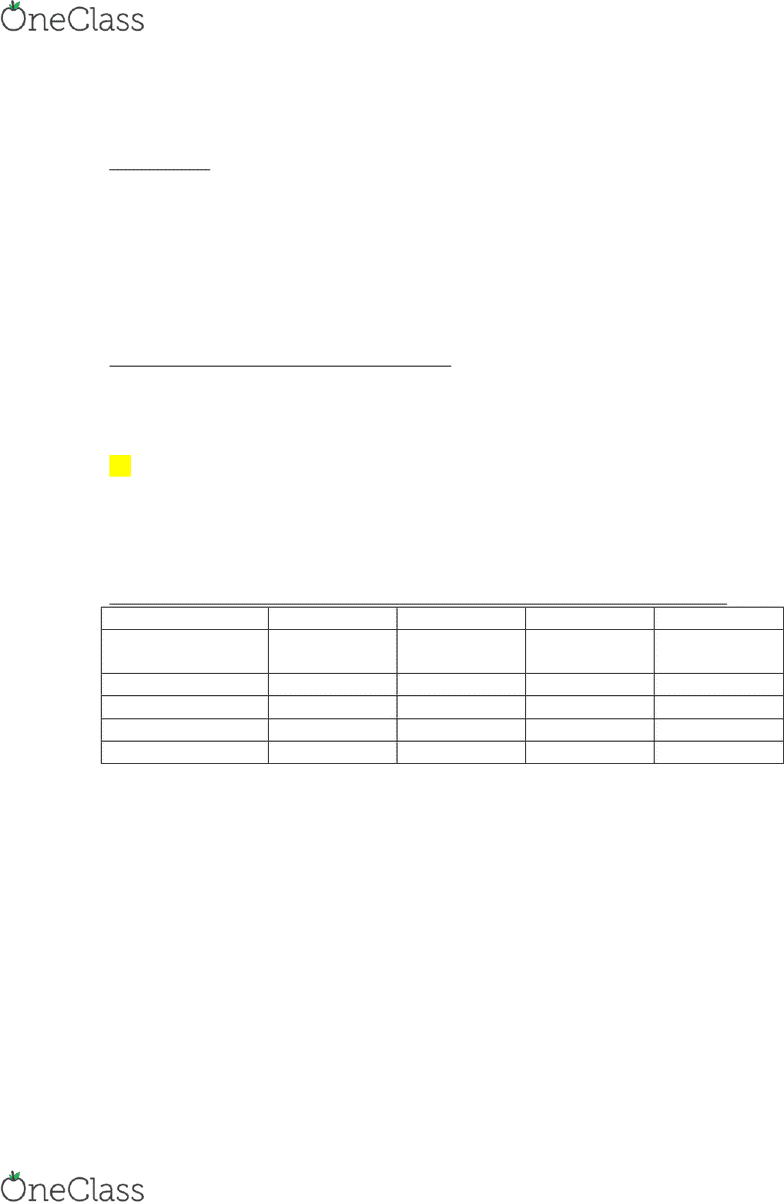# ACTG 2300 Midterm: Exam 1 In-Class Review

90 views5 pages
School
Department
Course

For unlimited access to Study Guides, a Grade+ subscription is required.Exam 1 Post Review
#2
Absorption Costing (used in calculating COGS)
DM = 150
DL = 320
VMOH = 40
o DON’T INCLUDE Var S&A
FMOH = 60,000 / 250 = 240
= 750 or A
Variable cost is always avoidable, so it is always relevant
#1
Sunk Cost: DEF: a cost that has already been incurred and does not change
Indirect Cost: we can never say whether a cost is direct or indirect if we do not know
what the cost object is
If we use the dish, it is indirect
If we use location, the cost can be directly traced
Product Cost: DEF: can be expensed and incurred in different periods can sit in the
balance sheet until we incur it
Period Cost: DEF: expensed (reported in the income statement) and incurred in the
same period
#1
High-Low Method
Cost estimation method
Use past to estimate the future
o If we assume normal conditions in future
Choose the HIGHEST BASED ON VOLUME, not the cost
A) UVC of Sales (EX: billboard doesn’t change with # of sales)
Includes manufacturing/product costs
(405,000 347,400) / (7,500 1,500) = \$38.4 / unit
Every unit we sell, we incur \$38.4 in sales
B) UVC of S&A (EX: sales commission changes with # of sales)
Includes only the period costs
(458,400 436,800) / (7,500 1,500) = \$14.4 / unit
find more resources at oneclass.com
find more resources at oneclass.com
Unlock document

This preview shows pages 1-2 of the document.
Unlock all 5 pages and 3 million more documents.

Already have an account? Log inEvery unit we sell, we incur \$14.4 in sales
C) Total Cost
TC = TFC + TVC
TFC = TC TVC
= 784,200 [(38.4+14.4)(6,000)] = 467,400
S&A AND Cost of sales fixed costs
TC = 52.8(volume) + 467,400
D) Total Estimated Cost for Volume = 6,200
TC = 52.8(6,200) + 467,400
TC = \$794,760
#2
Operating Income: income before interest, taxes, and dividends
TVC: changes with volume within a relevant range
A) Contribution Margin Income Statement (used internally, to make decisions)
Books
Electronics
Other
Total
+TR
(.37*700,000)
= 259,000
(.60*700,000)
= 420,000
(.03*700,000)
= 21,000
700,000
-TVC
215,600
394,800
29,400
639,800
=TCM
43,400
25,200
-8,400
60,200
-TFC
60,000
=OI
200
Prepared according to Variable Costing because we distinguish between
variable and fixed cost
B)
CM Ratio = TCM / TR = UCM / P = (P UVC) / P = (TR - TVC) / TR
BEP in \$ = TFC / CM Ratio
BEP in \$ = 60,000 / (60,200 / 700,000)
BEP in \$ = 697,674.41
C)
MOS in \$ = Total Act Rev BEP in \$
MOS in \$ = 700,000 697,674
find more resources at oneclass.com
find more resources at oneclass.com
Unlock document

This preview shows pages 1-2 of the document.
Unlock all 5 pages and 3 million more documents.

Already have an account? Log in

# Get access

Grade+
\$10 USD/m
Billed \$120 USD annually
Homework Help
Class Notes
Textbook Notes
40 Verified Answers
Study Guides
1 Booster Class